# Generating and Recognising Visual Charting Patterns

#### 2017-05-23

##
## Attaching package: 'rpatrec'
## The following object is masked from 'package:stats':
##
##     kernel
## Nonparametric Kernel Methods for Mixed Datatypes (version 0.60-2)
## [vignette("np_faq",package="np") provides answers to frequently asked questions]

Please note that this vignette is intended to be used alongside section 2 the Report submitted for MT4599, at the University of St Andrews, by Stephan Maier.

This Vignette is intended to demonstrate and test the capabilities of the R package RPatRec. In the following examples, all functions of the package will be used to allow comparison amongst their functionalities. Please refer to the following sections:

• Pattern Generation
• Real life data

# Pattern Generation

## Data without Noise

We can generate and plot a simple Head and Shoulders Pattern and an Inverse Head and Shoulders pattern, fully compliant with the definition given in the report using the following code:

a <- generator()
plot(a, type="l", ylab="price", xlab="Trading Days", main="HS")
b <- generator(plength=5,parts=c(0,15,30,50,70,85,100),sprd=c(0,-40,-20,-100,-20,-40,0))
plot(b, type="l", ylab="price", xlab="Trading Days", main = "Inverse HS")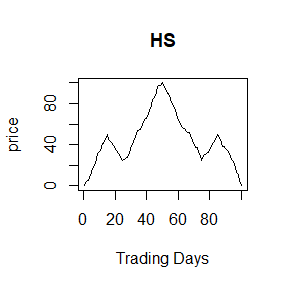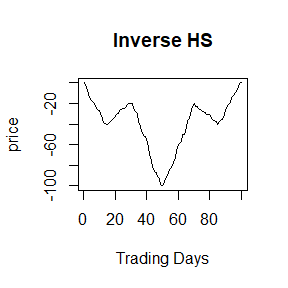Similarly, the other types of patterns are generated (their inverses follow logically and shall not be drawn):

#Double Tops
c <- generator(plength=3,parts=c(0,25,50,75,100),sprd=c(0,80,40,80,0))
plot(a, type="l", ylab="price", xlab="Trading Days", main="Double Tops")
#Rectangle Tops
d <- generator(plength=5,parts=c(0,20,40,50,60,80,100),sprd=c(0,80,40,80,40,80,0))
plot(d, type="l", ylab="price", xlab="Trading Days", main = "Rectangle Tops")
#Triangle Tops
e <- generator(plength=5,parts=c(0,15,30,50,70,85,100),sprd=c(0,100,10,60,20,30,0))
plot(e, type="l", ylab="price", xlab="Trading Days", main = "Triangle Tops")
#Broadening Tops
f <- generator(plength=5,parts=c(0,15,30,50,70,85,100),sprd=c(0,30,20,60,10,100,0))
plot(f, type="l", ylab="price", xlab="Trading Days", main = "Broadening Tops")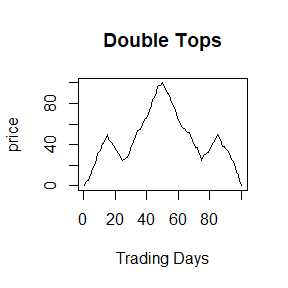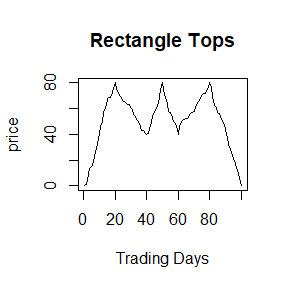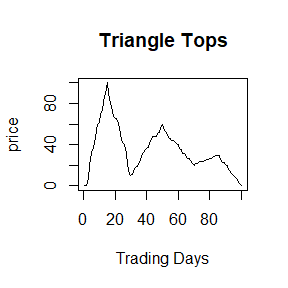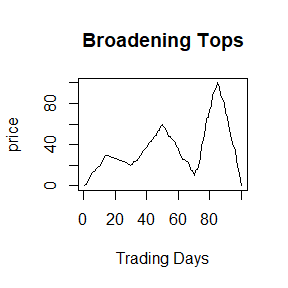With the right parameters, pattern generation is quite simple. Just to gain an understanding of how the recognition function works, and to test basic recognition of noise-less patterns that are generated with the perfect definition in mind.Hhere the sample output from analysing sample f:

interpret(f)
#> $EXT #>  1 0 1 0 1 #> #>$EXV
#>   30.00132  20.00096  60.00129  10.00088 100.00048
#>
#> $EXP #>  15 30 50 70 85 #> #>$HSP
#>  NA
#>
#> $BTPorTTP #>$BTPorTTP$BTOP #>  30.00132 20.00096 60.00129 10.00088 100.00048 #> #> #>$RTP
#>  NA
#>
#> $DTP #>  NA #> #>$RESULT
#>  TRUE

The output offers the user a list of the extrema, their values and their position in the time series date. Furthermore, for each recognised pattern, the maxima are output in a list of lists. (The name of the list is only created if a specific pattern (tops, bottoms) has been found and hence it is easy to check whether the elemnt exists in the data, in case the user wishes to further use the result). The following test is designed as a benchmark, it should yield 100% recognition rate if the software works well - however it may take a long time to compute:

#Number of runs
noruns <- 1
#define the pattern specifications:
specs <- list(c(0,15,30,50,70,85,100),c(0,15,30,50,70,85,100),c(0,20,40,50,60,80,100)
,c(0,25,50,75,100),c(0,15,30,50,70,85,100))
spreads <- list(c(0,40,20,100,20,40,0),c(0,30,20,60,10,100,0),c(0,80,40,80,40,80,0)
,c(0,80,40,80,0),c(0,100,10,60,20,30,0))
points <- c(5,5,5,3,5)
test1 <- vector()
#run the test for all specifications, 25 times each:
for(i in 1:5){
curspec <- specs[[i]]
cursprd <- spreads[[i]]
curp <- points [i]
success <- 0
for(j in 1:noruns){
curg <- generator(plength = curp, parts = curspec, sprd = cursprd)
cur <- interpret(curg)
#check whether the first recognised extreme is in order and whether the number of extremes is in order
k <- i
if(i==5)k <- 2
if(cur[[k+3]][] > cursprd*0.95 && cur[[k+3]][] < cursprd*1.05){
if(length(cur[])==curp)success <- success + 1
}
}
test1[i] <- success / noruns * 100
}
#the following line returns the recognition results in %
print(test1)
#>  100 100 100 100 100

This yields the 100% recognition rate, as expected.

## Data with Noise

For an initial example, we take a standard HS pattern, and then we add noise:

exp1 <- generator()
#white noise
exp2 <- noise(exp1,"white",5)
exp3 <- kernel(exp2,3)
plot(exp1, type="l", ylab="price", xlab="Trading Days", main="HS")
plot(exp2, type="l", ylab="price", xlab="Trading Days", main="HS with white noise, sd=5")
plot(exp3, type="l", ylab="price", xlab="Trading Days", main="HS with white noise, sd=5, smoothed with kernel regression")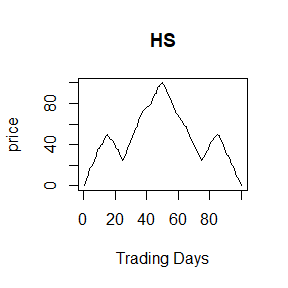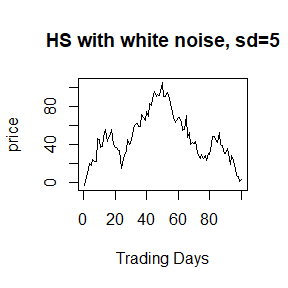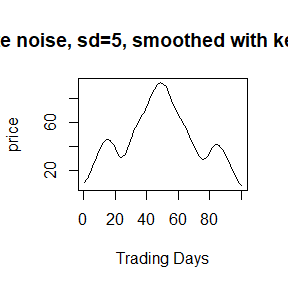The noisy pattern can no longer be easily recognised, the output of the interpret() function makes no sense (although it is likely that some pattern is recognised in the series of many extrema)

interpret(exp2)
#> $EXT #>  1 0 1 0 1 0 1 0 1 0 1 0 1 0 1 0 1 0 1 0 1 0 1 0 1 0 1 0 1 0 1 0 1 0 1 #>  0 1 0 1 0 1 0 1 0 1 0 1 0 1 0 1 0 1 0 #> #>$EXV
#>    19.611223  17.763069  23.533337  21.817179  45.891408  36.687691
#>    56.001625  42.924505  55.922345  36.476374  36.761117  14.978479
#>   44.910651  39.593126  62.395709  58.454802  71.146790  65.222200
#>   74.800419  69.285329  82.949857  81.387528  95.908953  91.070608
#>   92.344832  91.336590 105.089866  90.482325  95.401106  63.344242
#>   68.718770  54.902278  70.488418  47.627612  52.324154  39.758749
#>   41.713565  40.836305  43.034747  25.303011  29.368410  25.541638
#>   28.210011  23.493585  30.256768  29.277747  48.519395  42.017658
#>   52.147168  30.129951  35.296864  18.995959  28.600862   1.005777
#>
#> $EXP #>  4 5 6 7 9 11 14 15 18 20 21 24 28 29 34 35 37 39 40 41 42 43 45 #>  46 47 48 50 52 53 58 60 63 65 66 67 68 69 70 71 74 75 76 77 78 79 80 #>  83 85 86 90 91 93 94 99 #> #>$HSP
#> $HSP$HS
#>  45.89141 36.68769 56.00163 42.92451 55.92234
#>
#>
#> $BTPorTTP #>$BTPorTTP$TBOT #>  81.38753 95.90895 91.07061 92.34483 91.33659 #> #> #>$RTP
#>  NA
#>
#> $DTP #>  NA #> #>$RESULT
#>  TRUE

Smoothing the data can avoid this.The package provides the user with 5 methods for smoothing functions. Each will be tested in order to decide up to which level of noise it is capable of removing. To do so, the package provides the user with a testing function. A pattern is defined and generated, noise is added and gradually increased. The whole process is repeated n times, and each individual noise level is repeated k times.

### Kernel Regression

First define the number of test runs. This number is set delierately low now, so the package passes online testing. I recommend setting it to r = 5, s = 10 when experimenting with the code. For the package to pass online testing, they are set to r = 1, s = 5, to minimise computation time. This, however, negatively affect the quality of the plots.

#dummy variable for n
r <- 1
#dummy variable for m
s <- 3
a <- test.smoother(n=r,m=s,incr=0.5,max=50,smoother = kernel,bandwidth=1)
b <- test.smoother(n=r,m=s,incr=0.5,max=50,smoother = kernel,bandwidth=2)
c <- test.smoother(n=r,m=s,incr=0.5,max=80,smoother = kernel,bandwidth=3)
d <- test.smoother(n=r,m=s,incr=0.5,max=80,smoother = kernel,bandwidth=4)
#attach(mtcars)
#par(mfrow=c(2,2))
plot(a, type="l", ylab="% of patterns recognised", xlab="noise", main="Bandwidth = 1")
plot(b, type="l", ylab="% of patterns recognised", xlab="noise", main="Bandwidth = 2")
plot(c, type="l", ylab="% of patterns recognised", xlab="noise", main="Bandwidth = 3")
plot(d, type="l", ylab="% of patterns recognised", xlab="noise", main="Bandwidth = 4")
#detach(mtcars)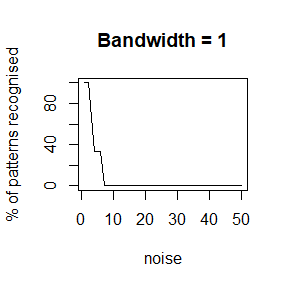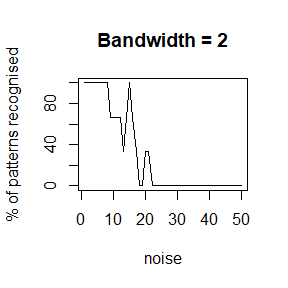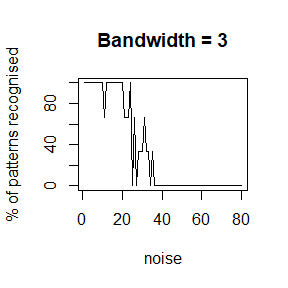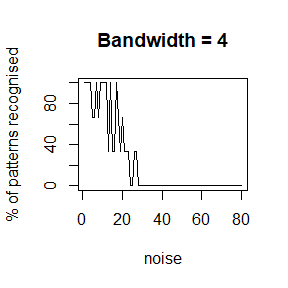a higher bandwidth seems to improve the recognition accross all values of noise. However, if the bandwidth is set too high:

a <- test.smoother(n=r,m=s,incr=0.5,max=80,smoother = kernel,bandwidth=5)
b <- test.smoother(n=r,m=s,incr=0.5,max=80,smoother = kernel,bandwidth=6)
c <- test.smoother(n=r,m=s,incr=0.5,max=80,smoother = kernel,bandwidth=7)
d <- test.smoother(n=r,m=s,incr=0.5,max=80,smoother = kernel,bandwidth=8)
#attach(mtcars)
#par(mfrow=c(2,2))
plot(a, type="l", ylab="% of patterns recognised", xlab="noise", main="Bandwidth = 5")
plot(b, type="l", ylab="% of patterns recognised", xlab="noise", main="Bandwidth = 6")
plot(c, type="l", ylab="% of patterns recognised", xlab="noise", main="Bandwidth = 7")
plot(d, type="l", ylab="% of patterns recognised", xlab="noise", main="Bandwidth = 8")
#detach(mtcars)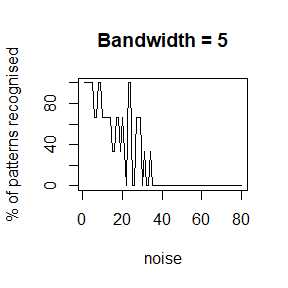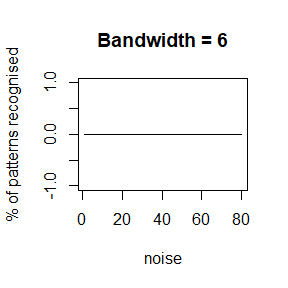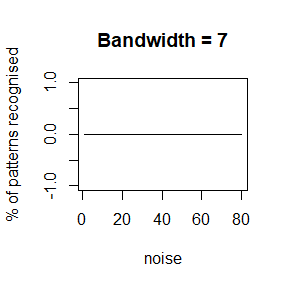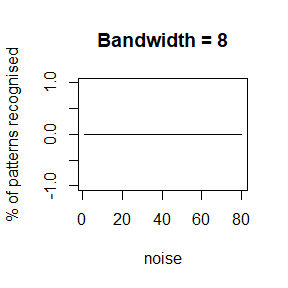### Savitzgy-Golay Filter

a <- test.smoother(n=r,m=s,incr=0.5,max=50,smoother = savgolay,width = 7)
b <- test.smoother(n=r,m=s,incr=0.5,max=50,smoother = savgolay,width = 8)
c <- test.smoother(n=r,m=s,incr=0.5,max=50,smoother = savgolay,width = 9)
d <- test.smoother(n=r,m=s,incr=0.5,max=50,smoother = savgolay,width = 10)
e <- test.smoother(n=r,m=s,incr=0.5,max=50,smoother = savgolay,width = 11)
f <- test.smoother(n=r,m=s,incr=0.5,max=50,smoother = savgolay,width = 12)
#attach(mtcars)
#par(mfrow=c(3,2))
plot(a, type="l", ylab="% of patterns recognised", xlab="noise", main="Width = 7, Degree = 2")
plot(b, type="l", ylab="% of patterns recognised", xlab="noise", main="Width = 8, Degree = 2")
plot(c, type="l", ylab="% of patterns recognised", xlab="noise", main="Width = 9, Degree = 2")
plot(d, type="l", ylab="% of patterns recognised", xlab="noise", main="Width = 10, Degree = 2")
plot(e, type="l", ylab="% of patterns recognised", xlab="noise", main="Width = 11, Degree = 2")
plot(f, type="l", ylab="% of patterns recognised", xlab="noise", main="Width = 12, Degree = 2")
#detach(mtcars)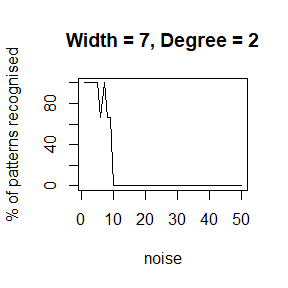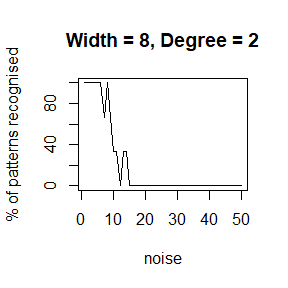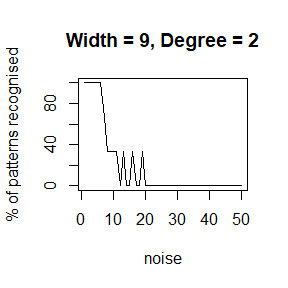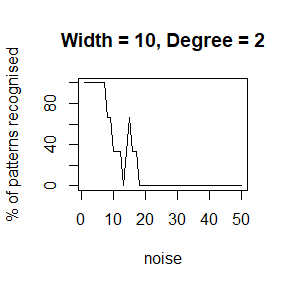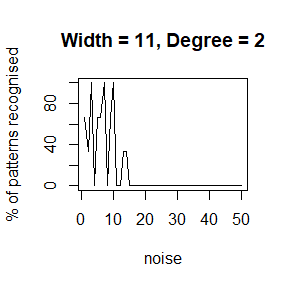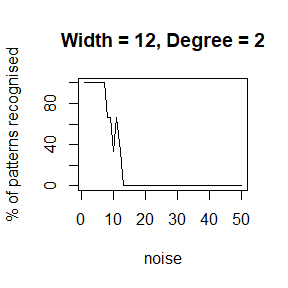a <- test.smoother(n=r,m=s,incr=0.5,max=50,smoother = savgolay, width = 10, degree = 1)
b <- test.smoother(n=r,m=s,incr=0.5,max=50,smoother = savgolay, width = 10, degree = 2)
c <- test.smoother(n=r,m=s,incr=0.5,max=50,smoother = savgolay, width = 10, degree = 3)
d <- test.smoother(n=r,m=s,incr=0.5,max=50,smoother = savgolay, width = 10, degree = 4)
#attach(mtcars)
#par(mfrow=c(2,2))
plot(a, type="l", ylab="% of patterns recognised", xlab="noise", main="Width = 10, Degree = 1")
plot(b, type="l", ylab="% of patterns recognised", xlab="noise", main="Width = 10, Degree = 2")
plot(c, type="l", ylab="% of patterns recognised", xlab="noise", main="Width = 10, Degree = 3")
plot(d, type="l", ylab="% of patterns recognised", xlab="noise", main="Width = 10, Degree = 4")
#detach(mtcars)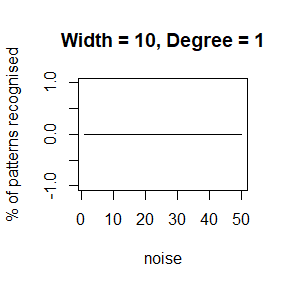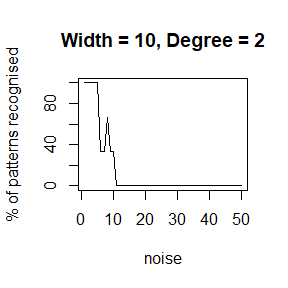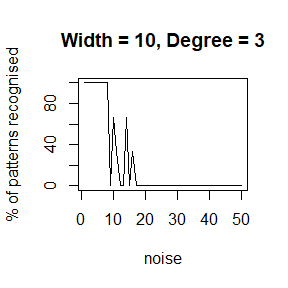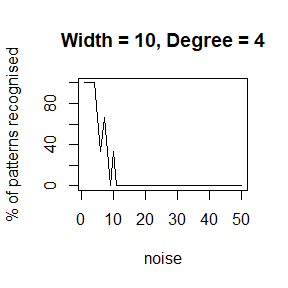### Moving Averages/Medians

a <- test.smoother(n=r,m=s,incr=0.5,max=50,smoother = mav, len = 5, method = "simple")
b <- test.smoother(n=r,m=s,incr=0.5,max=50,smoother = mav, len = 10, method = "simple")
c <- test.smoother(n=r,m=s,incr=0.5,max=50,smoother = mav, len = 15, method = "simple")
d <- test.smoother(n=r,m=s,incr=0.5,max=50,smoother = mav, len = 20, method = "simple")
#attach(mtcars)
#par(mfrow=c(2,2))
plot(a,type = "l", ylab="% of patterns recognised", xlab="noise", main="SMA, length = 5")
plot(b,type = "l", ylab="% of patterns recognised", xlab="noise", main="SMA, length = 10")
plot(c,type = "l", ylab="% of patterns recognised", xlab="noise", main="SMA, length = 15")
plot(d,type = "l", ylab="% of patterns recognised", xlab="noise", main="SMA, length = 20")
#detach(mtcars)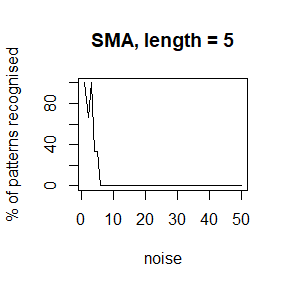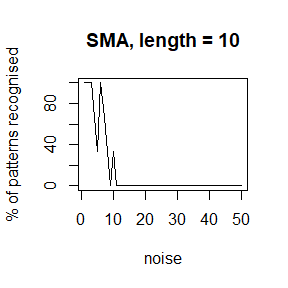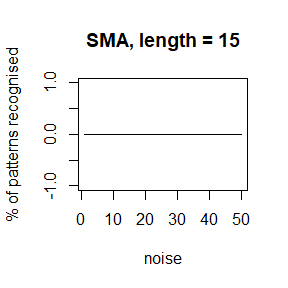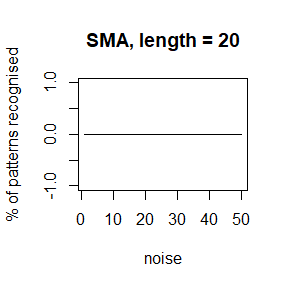#### Median

a <- test.smoother(n=r,m=s,incr=0.5,max=50,smoother = mav, len = 5, method = "median")
b <- test.smoother(n=r,m=s,incr=0.5,max=50,smoother = mav, len = 10, method = "median")
c <- test.smoother(n=r,m=s,incr=0.5,max=50,smoother = mav, len = 15, method = "median")
d <- test.smoother(n=r,m=s,incr=0.5,max=50,smoother = mav, len = 20, method = "median")
#attach(mtcars)
#par(mfrow=c(2,2))
plot(a,type = "l", ylab="% of patterns recognised", xlab="noise", main="Moving Median, length = 5")
plot(b,type = "l", ylab="% of patterns recognised", xlab="noise", main="Moving Median, length = 10")
plot(c,type = "l", ylab="% of patterns recognised", xlab="noise", main="Moving Median, length = 15")
plot(d,type = "l", ylab="% of patterns recognised", xlab="noise", main="Moving Median, length = 20")
#detach(mtcars)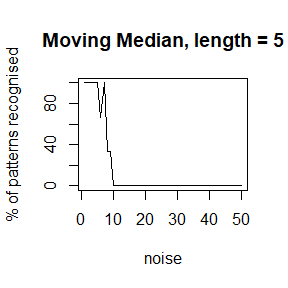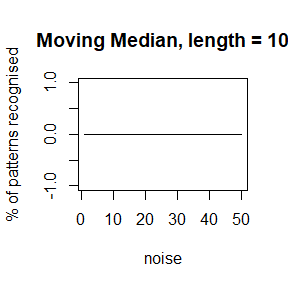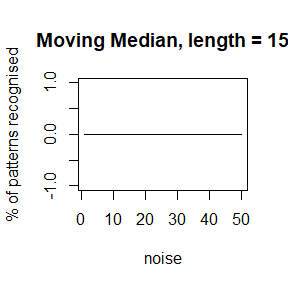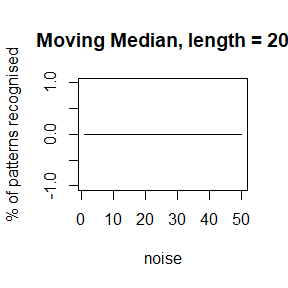#### Splines

a <- test.smoother(n=r,m=s,incr=0.5,max=50,smoother = splines, spar=0.1)
b <- test.smoother(n=r,m=s,incr=0.5,max=50,smoother = splines, spar=0.3)
c <- test.smoother(n=r,m=s,incr=0.5,max=60,smoother = splines, spar=0.5)
d <- test.smoother(n=r,m=s,incr=0.5,max=110,smoother = splines, spar=0.7)
#attach(mtcars)
#par(mfrow=c(2,2))
plot(a,type = "l", ylab="% of patterns recognised", xlab="noise", main="Smoothing Splines, spar = 0.1")
plot(b,type = "l", ylab="% of patterns recognised", xlab="noise", main="Smoothing Splines, spar = 0.3")
plot(c,type = "l", ylab="% of patterns recognised", xlab="noise", main="Smoothing Splines, spar = 0.5")
plot(d,type = "l", ylab="% of patterns recognised", xlab="noise", main="Smoothing Splines, spar = 0.7")
#detach(mtcars)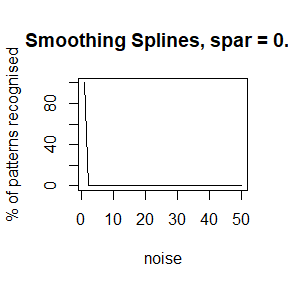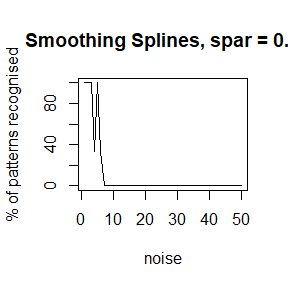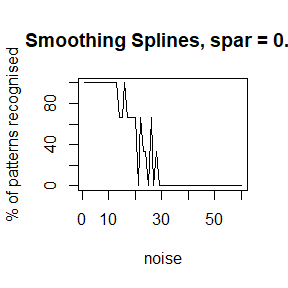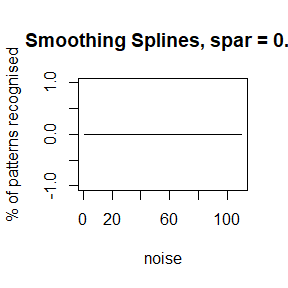#### LOESS

a <- test.smoother(n=r,m=s,incr=0.5,max=50,smoother = loess.rpatrec, span=0.1)
b <- test.smoother(n=r,m=s,incr=0.5,max=50,smoother = loess.rpatrec, span=0.2)
c <- test.smoother(n=r,m=s,incr=0.5,max=70,smoother = loess.rpatrec, span=0.3)
d <- test.smoother(n=r,m=s,incr=0.5,max=50,smoother = loess.rpatrec, span=0.4)
#attach(mtcars)
#par(mfrow=c(2,2))
plot(a,type = "l", ylab="% of patterns recognised", xlab="noise", main="LOESS, span = 0.1")
plot(b,type = "l", ylab="% of patterns recognised", xlab="noise", main="LOESS, span = 0.2")
plot(c,type = "l", ylab="% of patterns recognised", xlab="noise", main="LOESS, span = 0.3")
plot(d,type = "l", ylab="% of patterns recognised", xlab="noise", main="LOESS, span = 0.4")
#detach(mtcars)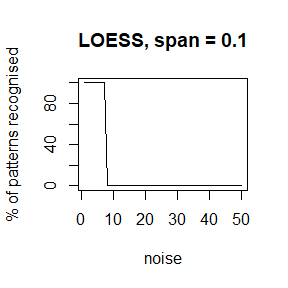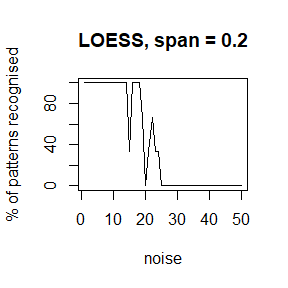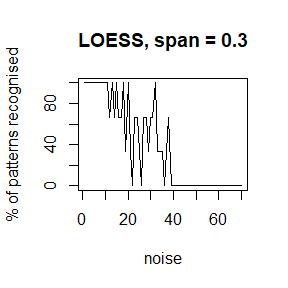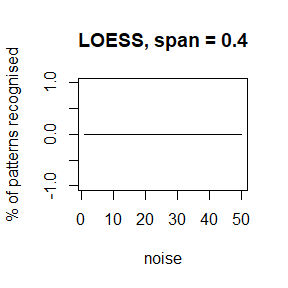## Red vs White Noise

a <- test.smoother(n=r,m=s,incr=0.5,max=50,smoother = kernel,ntype = "white", bandwidth=3)
b <- test.smoother(n=r,m=s,incr=0.5,max=50,smoother = kernel,ntype = "red", bandwidth=3)

#attach(mtcars)
#par(mfrow=c(2,2))
plot(a, type="l", ylab="% of patterns recognised", xlab="noise", main="White Noise")
plot(b, type="l", ylab="% of patterns recognised", xlab="noise", main="Red Noise")

#detach(mtcars)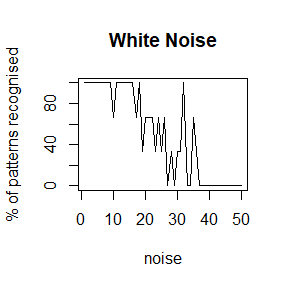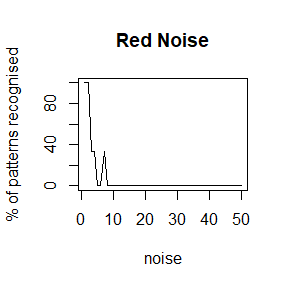## Real-life data

For reasons of practicality this has been split - please refer to the vignette Dissertation2